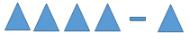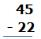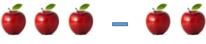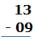# Test: Subtraction - 1

## 10 Questions MCQ Test Mathematics (Maths) for Class 1 | Test: Subtraction - 1

Description
This mock test of Test: Subtraction - 1 for Class 1 helps you for every Class 1 entrance exam. This contains 10 Multiple Choice Questions for Class 1 Test: Subtraction - 1 (mcq) to study with solutions a complete question bank. The solved questions answers in this Test: Subtraction - 1 quiz give you a good mix of easy questions and tough questions. Class 1 students definitely take this Test: Subtraction - 1 exercise for a better result in the exam. You can find other Test: Subtraction - 1 extra questions, long questions & short questions for Class 1 on EduRev as well by searching above.
QUESTION: 1

Solution:
QUESTION: 2

Solution:
QUESTION: 3

### Find the missing number : ___ - 2 = 0

Solution:
QUESTION: 4

What is 100-0

Solution:
QUESTION: 5

Find the missing number :

2 - ___ = 1

Solution:
QUESTION: 6Solution:
QUESTION: 7

Subtract the following numbers.Solution:
QUESTION: 8Solution:
QUESTION: 9

Subtract :

2 - 1

Solution:
QUESTION: 10

Subtract the following numbers.Solution:

= 13 - 9 = 4